# Analyzing New York City (NYC) High School Data¶

## 1. Introduction¶

In this project we are going to analyze SAT (Scholastic Aptitude Test) along with the demographics of each New York City High Schools. SAT is a test that high school seniors take every year in the US. It has three sections, each is worth 800 points. Colleges use the SAT scores to determine which student to admit. In the US educational system efficacy of these standardized tests are controversial. Our objective in this project is to find out if they are unfair to certain groups. New York City has significant immigrant population and is very diverse. So comparing demographic factors such as race, income, and gender with SAT scores is a good way of determining the authenticity of these tests. For e.g. if certain racial groups consistently perform better on the SAT, we can deduce it as unfair.

Link to the NYC data on high school SAT scores is here link

Following are the seven data-sets which we need to combine into a single dataset for the analysis:-

• SAT scores by school - SAT scores for each high school in New York City

• School attendance - Attendance information for each school in New York City

• Class size - Information on class size for each school

• AP test results - Advanced Placement (AP) exam results for each high school (passing an optional AP exam in a particular subject can earn a student college credit in that subject)

• Graduation outcomes - The percentage of students who graduated and other outcome information

• Demographics - Demographic information for each school

• School survey - Surveys of parents, teachers, and students at each school

## 2. Building a Single Dataset for Easy Analysis¶

### 2.1 Read in the CSV Data¶

Six of the data we need to analyze are in a single folder called data_files. Using pandas.read_csv() method we will store them in a dictionary called data for the ease of future analysis.

In :
import pandas as pd
import numpy
import re

data_files = [
"ap_2010.csv",
"class_size.csv",
"demographics.csv",
"hs_directory.csv",
"sat_results.csv"
]

data = {}

for f in data_files:
data[f.replace(".csv", "")] = d


### 2.2 Read in the Surveys Data¶

We read the survey data seperately as they are in the txt format unlike others which are in the csv format. We will club both the survey datasets all_survey and d75_survey into a single dataset using pd.concat() method. Then we will add this dataset in the data dictionary.

In :
all_survey = pd.read_csv("schools/survey_all.txt", delimiter="\t", encoding='windows-1252')
survey = pd.concat([all_survey, d75_survey], axis=0)

#change the dbn column case into capital
survey["DBN"] = survey["dbn"]

survey_fields = [
"DBN",
"rr_s",
"rr_t",
"rr_p",
"N_s",
"N_t",
"N_p",
"saf_p_11",
"com_p_11",
"eng_p_11",
"aca_p_11",
"saf_t_11",
"com_t_11",
"eng_t_11",
"aca_t_11",
"saf_s_11",
"com_s_11",
"eng_s_11",
"aca_s_11",
"saf_tot_11",
"com_tot_11",
"eng_tot_11",
"aca_tot_11",
]
survey = survey.loc[:,survey_fields]

#Add survey data into the data dictionary
data["survey"] = survey


DBN represents a School Identification Code (District Borough Number). Close observation of the data shows that the dataset class_size does not have a DBN column and dataset hs_directory has a dbn column, which needs to be converted into uppercase. In the class_size dataset DBN is just a combination of the CSD and SCHOOL CODE columns. Since the DBN is padded, the CSD portion of it always consists of two digits. That means we need to add a leading 0 to the CSD if the CSD is less than two digits long. We will create a function pad_csd() with number as a parameter. This function converts the number into a string and checks it's length. If the string is two digit long returns the string, if it is one digit long adds 0 in the front and returns it.

In :
#rename the dbn column in the hs_directory
data["hs_directory"]["DBN"] = data["hs_directory"]["dbn"]

string_representation = str(num)
if len(string_representation) > 1:
return string_representation
else:
return "0" + string_representation

#impliment the above function using Series.apply() method on CSD column

data["class_size"]["DBN"] = data["class_size"]["padded_csd"] + data["class_size"]["SCHOOL CODE"]


### 2.4 Converting Columns into Numeric¶

In the sat_results dataset, three columns SAT Math Avg. Score, SAT Critical Reading Avg. Score, and SAT Writing Avg. Score totals up the SAT scores for the different sections of the exam. Working with a single number rather than a three makes it easier to correlate scores with demographic factors. These three columns are in string format, so we need to convert them into numeric type before adding them up.

Next, we will parse the latitude and longitude coordinates for each school. This enables us to map the schools and uncover any geographic patterns in the data. The coordinates are currently in the text field Location 1 in the hs_directory dataset. We will create two functions one for returning latitude called find_lat() & another for finding longitude called find_lon(). We will use regular expressions to pull out the latitude and longitude, then use string methods split() and replace() to extract each coordinate. Convert them to numeric type using pd.to_numeric() method.

In :
#convert the following three column into numeric type using pd.to_numeric() method & add them up
cols = ['SAT Math Avg. Score', 'SAT Critical Reading Avg. Score', 'SAT Writing Avg. Score']
for c in cols:
data["sat_results"][c] = pd.to_numeric(data["sat_results"][c], errors="coerce")

data['sat_results']['sat_score'] = data['sat_results'][cols] + data['sat_results'][cols] + data['sat_results'][cols]

#create find_lat() & find_lon() functions to extract latitude & longitude seperately
def find_lat(loc):
coords = re.findall("$.+, .+$", loc)
lat = coords.split(",").replace("(", "")
return lat

def find_lon(loc):
coords = re.findall("$.+, .+$", loc)
lon = coords.split(",").replace(")", "").strip()
return lon

#using Series.apply() method impliment the above functions & extract the latitudes and longitudes
data["hs_directory"]["lat"] = data["hs_directory"]["Location 1"].apply(find_lat)
data["hs_directory"]["lon"] = data["hs_directory"]["Location 1"].apply(find_lon)

#convert the lat & lon columns into numeric type using pd.to_numeric() method
data["hs_directory"]["lat"] = pd.to_numeric(data["hs_directory"]["lat"], errors="coerce")
data["hs_directory"]["lon"] = pd.to_numeric(data["hs_directory"]["lon"], errors="coerce")


### 2.5 Condensing the Datasets¶

We will seperate the class_size dataset from data dictionary for further analysis. Since we are only interested in high schools, we need to filter the GRADE column by selecting only 09-12 grades. In PROGRAM TYPE column GEN ED is the largest category, so we will only filter it. The CORE SUBJECT column has four subjects 'ENGLISH', 'MATH', 'SCIENCE', 'SOCIAL STUDIES'. Each has unique DBN values. Using the df.groupby() method, we will split this dataframe into four separate groups. Then we will compute the average for the AVERAGE CLASS SIZE column in each of the four groups using the agg() method. After we are done with the above operations we will include the class_size dataset back into data dictionary.

In demographics dataset we are only interested in the most recent school year. i.e. 20112012. We will filter this year from the schoolyear column. This will also match SAT results data.

The Demographic and Cohort columns in the graduation dataset prevent DBN from being unique. A Cohort appears to refer to the 'year' the data represents, and the Demographic appears to refer to a 'specific demographic group'. We will pick data from the most recent Cohort available, which is 2006. We also want data from the full cohort, so we'll only pick rows where Demographic is Total Cohort.

In :
#from the class_size select grades '09-12' & program type 'GEN ED'
class_size = data["class_size"]
class_size = class_size[class_size["GRADE "] == "09-12"]
class_size = class_size[class_size["PROGRAM TYPE"] == "GEN ED"]

#calculate the average DBN value for each subject using Series.groupby().agg() method
class_size = class_size.groupby("DBN").agg(numpy.mean)
class_size.reset_index(inplace=True)
data["class_size"] = class_size

#select 20112012 schoolyear from the demographics dataset
data["demographics"] = data["demographics"][data["demographics"]["schoolyear"] == 20112012]

#filter year 2006 from Cohort column & total Cohort from the Demographics column in graduation dataset


### 2.6 Converting AP Scores to Numeric¶

Advanced Placement (AP) test is an exam high school students take before applying to college. There are several AP exams, each corresponding to a school subject. High school students who earn scores 3 and above (AP exams have 1 to 5 scale) may receive college credit. It will be interesting to find out whether AP exam scores are correlated with SAT scores across high schools. For this first we need to convert the AP exam scores in the ap_2010 data set to numeric values.

In :
#convert the columns related to AP exams in ap_2010 dataset to numeric value
cols = ['AP Test Takers ', 'Total Exams Taken', 'Number of Exams with scores 3 4 or 5']

for col in cols:
data["ap_2010"][col] = pd.to_numeric(data["ap_2010"][col], errors="coerce")


### 2.7 Combining the Datasets¶

Since we want our final dataframe to have the same DBN values as the original sat_results dataframe. For this purpose we will use pandas.dataframe.merge() method with keyword arguments how='left' and on='DBN'. First, we'll assign sat_results to the variable combined. Then, we will merge the other dataframes with combined.

In :
#Create a variable combined with just sat_results from the data then merge
#remaining dataframes

combined = data["sat_results"]

combined = combined.merge(data["ap_2010"], on="DBN", how="left")

to_merge = ["class_size", "demographics", "survey", "hs_directory"]

for m in to_merge:
combined = combined.merge(data[m], on="DBN", how="inner")

#fill the null values with mean value using df.fillna() method &
#any remaining null value with 0 value
combined = combined.fillna(combined.mean())
combined = combined.fillna(0)


### 2.8 Adding a School District Column for Mapping¶

Finally, we are done with cleaning and combining the data. An interesting way of analyzing it is to map the statistics out on a school district level. Adding a column to the data which specifies the school district will help us achieve this. School district is just the first two characters of the DBN. We will write a function get_first_two_chars() inorder to accomplish this.

In :
#define a function to extract first two characters of DBN
def get_first_two_chars(dbn):
return dbn[0:2]

#Apply the above function on DBN columnm & store it in a new column called school_dist
combined["school_dist"] = combined["DBN"].apply(get_first_two_chars)


## 3. Finding Correlations¶

Correlations depicts how closely any two columns are related. We will use pd.df.corr() method to find the correlation between different columns in our dataset. The method returns a new dataframe where the index for each column and row is the name of a column in the original dataset.

In :
#create a new dataset correlations with correlation values between the columns
correlations = combined.corr()

#filter just the sat_score in the correlation dataset & print the values
correlations = correlations["sat_score"]
print(correlations)

SAT Critical Reading Avg. Score    0.986820
SAT Math Avg. Score                0.972643
SAT Writing Avg. Score             0.987771
sat_score                          1.000000
AP Test Takers                     0.523140
...
priority08                              NaN
priority09                              NaN
priority10                              NaN
lat                               -0.121029
lon                               -0.132222
Name: sat_score, Length: 67, dtype: float64


From the above data we make following observations:-

• The SAT Critical Reading Avg. Score, SAT Math Avg. Score, SAT Writing Avg. Score, and sat_score strongly correlate with sat_score.
• Column total_enrollment has a positive correlation with sat_score. This implies that the larger schools do better on SAT in condradiction to assumption that smaller schools where students receive more attention get higher scores. Other similar columns total_students, N_s, N_p, N_t, AP Test Takers, Total Exams Taken, and NUMBER OF SECTIONS correlate similarly.
• Both the percentage of females female_per and number of females female_num at a school correlate positively with SAT score, whereas the percentage of males male_per and the number of males male_num correlate negatively. This indicates that girls do better in SAT than boys.
• Teacher and student ratings of school safety saf_t_11, and saf_s_11 correlate with sat_score.
• Student ratings of school academic standards aca_s_11 correlate positively with sat_score, but this does not apply to the ratings from teachers and parents aca_p_11 and aca_t_11.
• There is significant racial inequality in SAT scores, i.e. correlation is highest for white_per and then for asian_per, both being positive. But black_per, hispanic_per correlates negatively with the latter being lowest.
• The percentage of English language learners at the school ell_percent, frl_percent has a strong negative correlation with SAT score.

## 4. Plotting Survey Correlations¶

We will further analyze different survey fields (survey_fields) with sat_score. First we will find the correlation between these fields and sat_score and then plot them using bar plot. This will give us a clear picture of how the correlation values of these various survey fields varies with sat_score.

In :
%matplotlib inline
import matplotlib.pyplot as plt

# Remove DBN since it's a unique identifier, not a useful numerical value for correlation.
survey_fields.remove("DBN")
print(correlations[survey_fields])

#make a bar plot of correlations between these fields & sat_score
correlations[survey_fields].plot.bar()
plt.xlabel('Survey Fileds')
plt.ylabel('Sat Score')
plt.title('Correlation Bar Plot')
plt.show()

rr_s          0.232199
rr_t         -0.023386
rr_p          0.047925
N_s           0.423463
N_t           0.291463
N_p           0.421530
saf_p_11      0.122913
com_p_11     -0.115073
eng_p_11      0.020254
aca_p_11      0.035155
saf_t_11      0.313810
com_t_11      0.082419
eng_t_11      0.036906
aca_t_11      0.132348
saf_s_11      0.337639
com_s_11      0.187370
eng_s_11      0.213822
aca_s_11      0.339435
saf_tot_11    0.318753
com_tot_11    0.077310
eng_tot_11    0.100102
aca_tot_11    0.190966
Name: sat_score, dtype: float64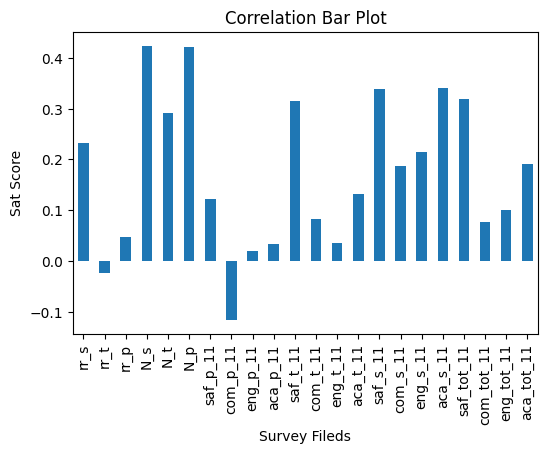Above graph depicts the correlation betweensat_scoreandsurvey_fields. Looking at the graph and the correlation values we make following observations:-

• Except com_p_11 (communication score based on parent responses) and rr_t (teacher response rate) all other survey fileds have positive correlation with sat_score.
• Highest of all is N_s (number of student respondents) and N_p (number of parent respondents) with ~0.42.
• Next highest are saf_s_11 (safety and respect score based on student responses) and aca_s_11 (academic expectations score based on student responses) at ~0.34.
• The third highest are saf_t_11 (safety and respect score based on teacher responses) and saf_tot_11 (safety and respect total score) at ~0.31.

### 4.1 Exploring Safety and SAT Scores¶

Above analysis indicates that saf_s_11 (how students perceive safety) and saf_tot_11 (total safety) correlation values (verses SAT Scores) are ~0.34 and ~0.31 respectively. In here we will dig deeper into this relationship and figure out which schools have low safety scores.

First we will plot saf_s_11 vs sat_score from the combined dataset.

In :
combined.plot.scatter(y = 'sat_score', x = 'saf_s_11')
plt.ylabel('SAT Score')
plt.xlabel('Student Safety')
plt.title("Student Safety vs SAT Score")
plt.show()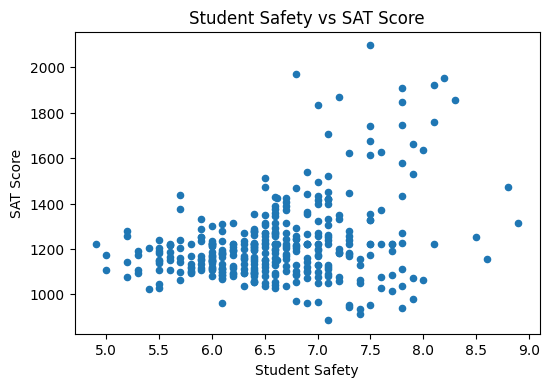From the above scatter plot of Student Safety vs SAT Score, we observe that there is a very gradual but steady increase in the SAT score with increase in the Student safety. Even if it does not apply in all the cases, overall increase is aparent. There is an accumulation of data points in the region 5.5 to 7.5 in students safety and between 1000 & 1500 in SAT Score. These data points show that with the same safety value, SAT scores can be different for different schools indicating that in some cases there is no relation between students safety and SAT score.

Below we will compute the average Safety Score (we will take total safety values saf_tot_11 for this purpose) for each borough in the New York city. New York city has been devided into 5 boroughs. These are listed below:-

• Brooklyn
• Manhattan
• Queens
• Staten Island
• The Bronx

Here we have a column boro in the dataframe, which has relevant boroughs. Then using df.pivot_table() method we will calculate the mean safety values for each borough.

In :
#apply value_counts() method to calculate the number of each borough
combined['boro'].value_counts().sort_values(ascending=False)

Out:
Brooklyn         106
Bronx             93
Manhattan         90
Queens            64
Staten Island     10
Name: boro, dtype: int64
In :
#apply df.pivot_table() method to calculate the average safety score for each borough
combined.pivot_table(index='boro', values='saf_tot_11', aggfunc= 'mean')

Out:
saf_tot_11
boro
Bronx 7.322581
Brooklyn 7.129245
Manhattan 7.473333
Queens 7.387500
Staten Island 7.200000

The above table shows the average safety values for all the five boroughs. Average safety value for each borough is roughly at ~7. The highest among them is Manhattan with 7.47 of average safety value. Least of them is Brooklyn at 7.13.

## 5. Exploring Race and SAT Scores¶

In this section we are going to explore the SAT Scores of various Races. There are four races listed in the data. These are Whites, Asians, Blacks and Hispanics. Below we will look into the correlation values for different races with SAT Score.

In :
#print the correlation value between different races and sat scores
print('whites vs sat score: ', correlations['white_per'])
print('asians vs sat score: ', correlations['asian_per'])
print('blacks vs sat score: ', correlations['black_per'])
print('hispanics vs sat score: ', correlations['hispanic_per'])

whites vs sat score:  0.6207177638526802
asians vs sat score:  0.5707302200214872
blacks vs sat score:  -0.2841394684581478
hispanics vs sat score:  -0.39698493078147196


From the above calculation we observe that there are two negative correlations one for blacks and other for hispanics. Below we will build a bar plot in order to look at the better picture of correlation value.

In :
#make a bar plot for all the races with sat score
correlations[['white_per', 'asian_per', 'black_per', 'hispanic_per']].plot(x='sat_score', kind='bar')
plt.title('Race vs SAT Score')
plt.ylabel('SAT Score')
plt.xlabel('Race')
plt.xticks(rotation=45)

Out:
(array([0, 1, 2, 3]), <a list of 4 Text major ticklabel objects>)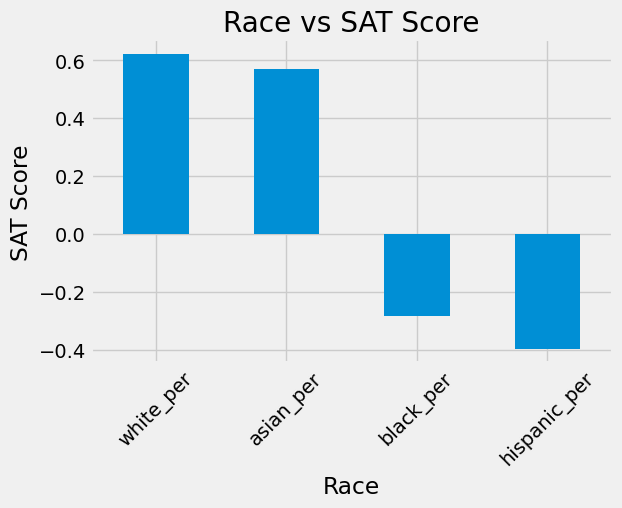From the above table it is very clear the difference between correlation value of different races with sat score. First highest is for whites at ~ 62% and second highsest is for Asians, slightly lower at ~ 57%. But for blacks and hispanics, it is negative at ~ -28% and ~ -40% respectively.

Below we will explore more about hispanic percentage and SAT Score. We want to know the schools with high percentage of hispanics and low SAT Scores. We are going to make a scatter plot for hispanic_per and sat_score.

In :
#build a scatter plot for Hispanics vs SAT score
combined.plot.scatter(x='hispanic_per', y='sat_score')
plt.xlabel('Hispanic percentage')
plt.ylabel('SAT Score')
plt.title('Hispanic percentage vs SAT Score')

Out:
Text(0.5, 1.0, 'Hispanic percentage vs SAT Score')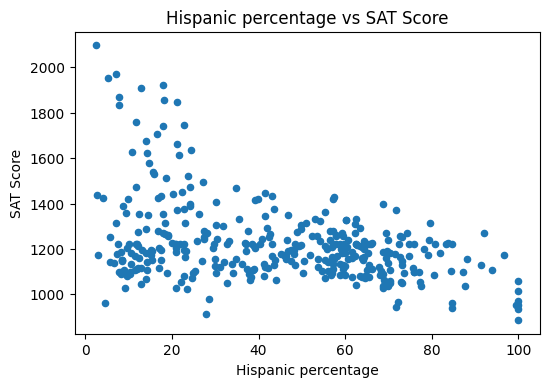The negative trend between the SAT Score and Hispanic Percentage is very clear from the above graph. At schools with low percentage of hispanics the SAT Score is considerably higher. With increase in the percentage of hispanics, SAT Score decreases exponentially. Higher the percentage of hispanics lower the SAT Score.

Below we will explore the schools with higher hispanics percentage, i.e. above 95%.

In :
hispanic_95 = combined[combined['hispanic_per']> 95.0]
hispanic_95[['SCHOOL NAME', 'hispanic_per']]

Out:
SCHOOL NAME hispanic_per
44 MANHATTAN BRIDGES HIGH SCHOOL 99.8
82 WASHINGTON HEIGHTS EXPEDITIONARY LEARNING SCHOOL 96.7
89 GREGORIO LUPERON HIGH SCHOOL FOR SCIENCE AND M... 99.8
125 ACADEMY FOR LANGUAGE AND TECHNOLOGY 99.4
141 INTERNATIONAL SCHOOL FOR LIBERAL ARTS 99.8
176 PAN AMERICAN INTERNATIONAL HIGH SCHOOL AT MONROE 99.8
253 MULTICULTURAL HIGH SCHOOL 99.8
286 PAN AMERICAN INTERNATIONAL HIGH SCHOOL 100.0

There are 8 schools in the list which have above 95% of hispanics. In these schools atleast 80% of students are economically disadvantaged, in some schools 100% students are economically disadvantaged. These schools have very low national rankings ranging from above 1000 and in some cases even closer to 10000. New York High Schools rankings is also lower for these schools, in some cases above 100 and in others closer to 800. The minority enrolment in these schools are almost 100%.

Below we will explore the schools with percentage of hispanics less than 10% and average SAT Score greater than 1800.

In :
hisp_10 =  combined[(combined['hispanic_per']<10.0) & (combined['sat_score']>1800)]
hisp_10[['SCHOOL NAME', 'sat_score', 'hispanic_per']]

Out:
SCHOOL NAME sat_score hispanic_per
37 STUYVESANT HIGH SCHOOL 2096.0 2.4
151 BRONX HIGH SCHOOL OF SCIENCE 1969.0 7.2
187 BROOKLYN TECHNICAL HIGH SCHOOL 1833.0 7.9
327 QUEENS HIGH SCHOOL FOR THE SCIENCES AT YORK CO... 1868.0 7.9
356 STATEN ISLAND TECHNICAL HIGH SCHOOL 1953.0 5.3

There are only 5 schools in the list which have less than 10% of hispanics and more than 1800 SAT Scores. Among these schools minority enrolment is comparatively lesser than the list with high hispanic percentage and lower percentage of economically disadvantaged students highest being ~ 60%. The national rankings of these schools are very good. Highest being 22 and lowest being 44. New York High School Rankings are also great, all five are within 10. Among the above two lists one more point to be noted is number of full-time teachers. This number is considerably higher for schools with lower hispanic percentage compared to higher hispanic percentage.

## 6. Exploring Gender and SAT Scores¶

There are two columns in the dataframe which indicate the percentage of gender.

• male_per (male percentage)
• female_per (female percentage)

In here we will investigate the correlation between these two columns and the SAT score. First we will plot the correlation and study the graph.

In :
#print the correlation between sat score and genders
print('male percentage Vs SAT Score: ', correlations['male_per'])
print('female percentage Vs SAT Score: ', correlations['female_per'])

male percentage Vs SAT Score:  -0.11206243686028577
female percentage Vs SAT Score:  0.11210773942203284

In :
#make a bar plot of gender vs sat score
correlations[['male_per', 'female_per']].plot(x='sat_score', kind='bar')
plt.xlabel('Gender Percentage')
plt.ylabel('SAT Score')
plt.title('Gender Percentage Vs SAT Score')
plt.xticks(rotation=45)

Out:
(array([0, 1]), <a list of 2 Text major ticklabel objects>)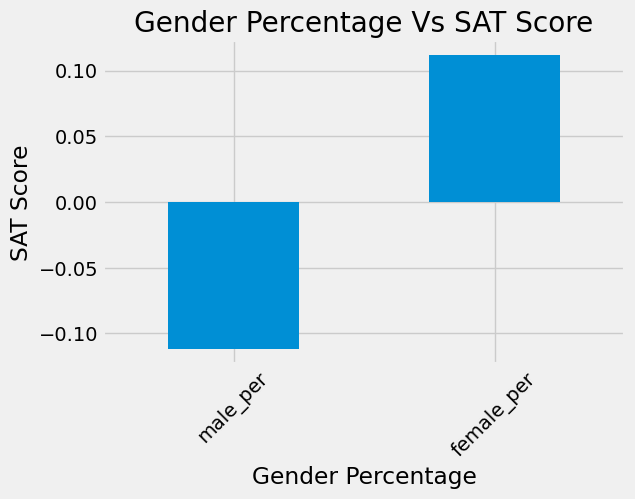Above analysis shows that the male percentage has a negative correlation and female percentage has a positive correlation with SAT score. The correlation values being -0.112 and 0.112 for male and female respectively. The contrast is very much evident from the above bar plot. We will further investigate the relation between SAT scores, female percentage and relevant schools. We will make a scatter plot and observe if there is any pattern between SAT score and female percentage.

In :
#make a scatter plot of the female percentage and sat score
combined.plot.scatter(x='female_per', y='sat_score')
plt.xlabel('Female percentage')
plt.ylabel('SAT Score')
plt.title('Female percentage vs SAT Score')

Out:
Text(0.5, 1.0, 'Female percentage vs SAT Score')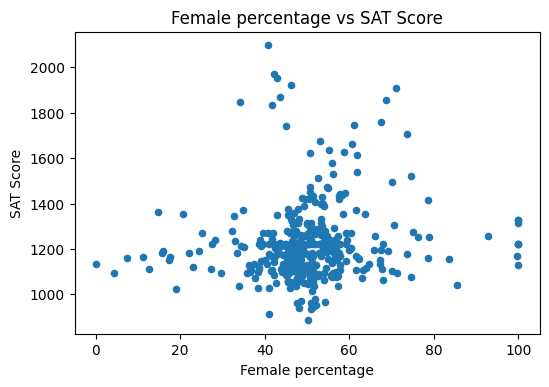There is no clear pattern between the SAT Score and Female percentage. SAT score is low where the female percentage is either lower (below 40%) and also where the female percentage is higher (above 80%). There is a cluster between 40% and 60%. There are two peaks, one at ~ 40% with SAT score of ~2200 and another at ~ 70% with SAT score of ~ 1900. Further we will explore more about schools with female percentage > 60 and SAT score > 1700.

In :
#consider only data with female percentage abobe 60 and sat score above 1700
female_60 = combined[(combined['female_per'] > 60) & (combined['sat_score'] > 1700)]
female_60[['SCHOOL NAME','sat_score', 'female_per']]

Out:
SCHOOL NAME sat_score female_per
5 BARD HIGH SCHOOL EARLY COLLEGE 1856.0 68.7
26 ELEANOR ROOSEVELT HIGH SCHOOL 1758.0 67.5
60 BEACON HIGH SCHOOL 1744.0 61.0
61 FIORELLO H. LAGUARDIA HIGH SCHOOL OF MUSIC & A... 1707.0 73.6
302 TOWNSEND HARRIS HIGH SCHOOL 1910.0 71.1

There are total 5 schools with female percentage higher than 60 and SAT score more than 1700. In the above list only two of the schools Townsend Harris High School (New York High School Ranking is 1) and Eleanor Roosevelt High School (New York High School Ranking is 11) have national ranking below 100. Bard High School Early College with very poor national ranking (~5000) and state ranking (~400) has an average SAT score of 1856 which is impressive.

## 7. Exploring AP Scores and SAT Scores¶

In here we are going to explore the relationship between Advanced Placement (AP) scores and SAT scores. It is reasonable to assume that the students who took AP exams would be highly correlated with school's SAT score. total_enrollment column is highly correlated with sat_score column, this could lead to a bias. So we will take AP percentage of students by dividing AP Test Takers column by total_enrollment column. We will plot this new column ap_per and sat_score and analyze the graph.

In :
#divide AP test takers column by total enrollment and name the new column ap_per
combined['ap_per'] = combined['AP Test Takers ']/combined['total_enrollment']
combined['ap_per']

Out:
0      0.305756
1      0.098985
2      0.031773
3      0.351577
4      0.158091
...
358    0.077558
359    0.297301
360    0.190955
361    0.026616
362    0.073593
Name: ap_per, Length: 363, dtype: float64
In :
#make a scatter plot of ap_per vs sat_score
combined.plot.scatter(x='ap_per', y='sat_score')
plt.xlabel('AP percentage')
plt.ylabel('SAT Score')
plt.title('AP percentage vs SAT Score')

Out:
Text(0.5, 1.0, 'AP percentage vs SAT Score')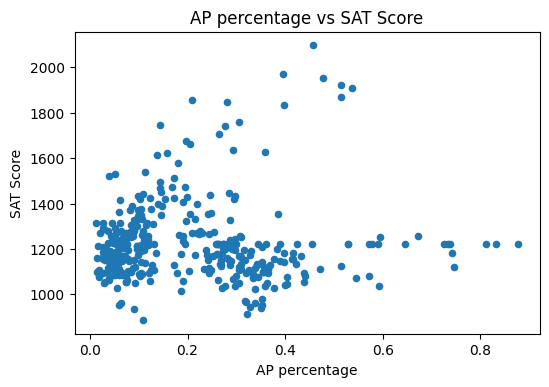The graph of AP percentage and SAT score looks like a C-curve. There are two clusters at the lower left side of the graph. Highest SAT score is recorded with AP percentage between 0.4 and 0.6. As the AP percentage reduces SAT score also reduces as we can see at the left upper side of the graph. At the lower part of the graph SAT score is more or less same against AP percentage.

## 8. Exploring Class Size and SAT Scores¶

In this section we will find more about how average class size is related to SAT score. Let us look at the correlation value between these two columns.

In :
#print the correlation value between average class size and sat_score
print("average class size vs sat_score: ",correlations["AVERAGE CLASS SIZE"])

average class size vs sat_score:  0.3810143308095523


There is a strong correlation between average class size and SAT score. Further we will build a scatter plot and observe the graph.

In :
#make a scatter plot between average class size and sat score
combined.plot.scatter(x='AVERAGE CLASS SIZE', y='sat_score')
plt.xlabel("Average Class Size")
plt.ylabel("SAT Score")
plt.title("Average Class Size vs SAT Score")

Out:
Text(0.5, 1.0, 'Average Class Size vs SAT Score')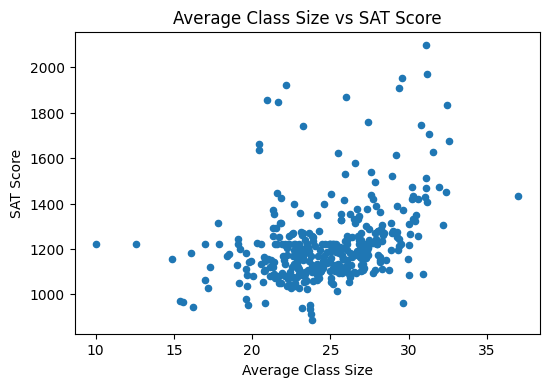The scatter plot shows that there is an exponential increase between SAT Score and average class size. There is a cluster between 20 and 30 in the average class size, and between 1000 and 1300 in the SAT Score. Even if the points are scattered around the pattern is very clear.

## 9. Exploring Least Expensive Neighborhoods and Best Schools¶

In this section we will look for the least expensive neighborhoods with best schools. For this we need to import a csv datafile containing the details of neighborhoods and house prices. The file link has been given in the introduction (demographics for each school). This file was first downloaded into the local computer and then uploaded to the dataquest repository.

In :
#read the property.csv file using pd.read_csv() method and look at the first few rows

Out:
dbn school_name borough building_code phone_number fax_number grade_span_min grade_span_max expgrade_span_min expgrade_span_max ... priority08 priority09 priority10 Location 1 Community Board Council District Census Tract BIN BBL NTA
0 27Q260 Frederick Douglass Academy VI High School Queens Q465 718-471-2154 718-471-2890 9.0 12 NaN NaN ... NaN NaN NaN 8 21 Bay 25 Street\nFar Rockaway, NY 11691\n(4... 14.0 31.0 100802.0 4300730.0 4.157360e+09 Far Rockaway-Bayswater ...
1 21K559 Life Academy High School for Film and Music Brooklyn K400 718-333-7750 718-333-7775 9.0 12 NaN NaN ... NaN NaN NaN 2630 Benson Avenue\nBrooklyn, NY 11214\n(40.59... 13.0 47.0 306.0 3186454.0 3.068830e+09 Gravesend ...
2 16K393 Frederick Douglass Academy IV Secondary School Brooklyn K026 718-574-2820 718-574-2821 9.0 12 NaN NaN ... NaN NaN NaN 1014 Lafayette Avenue\nBrooklyn, NY 11221\n(40... 3.0 36.0 291.0 3393805.0 3.016160e+09 Stuyvesant Heights ...
3 08X305 Pablo Neruda Academy Bronx X450 718-824-1682 718-824-1663 9.0 12 NaN NaN ... NaN NaN NaN 1980 Lafayette Avenue\nBronx, NY 10473\n(40.82... 9.0 18.0 16.0 2022205.0 2.036040e+09 Soundview-Castle Hill-Clason Point-Harding Par...
4 03M485 Fiorello H. LaGuardia High School of Music & A... Manhattan M485 212-496-0700 212-724-5748 9.0 12 NaN NaN ... NaN NaN NaN 100 Amsterdam Avenue\nNew York, NY 10023\n(40.... 7.0 6.0 151.0 1030341.0 1.011560e+09 Lincoln Square ...

5 rows × 64 columns

In :
#rename the *dbn* column name to *DBN* and merge it into the main dataset
prop_data['DBN'] = prop_data['dbn']
prop_data['Price_$'] = prop_data['BIN'] prop_data['Neighborhood'] = prop_data['NTA'] combined = combined.merge(prop_data, on="DBN", how="inner")  In the dataset prop_data the property prices are listed in the column Price_$. First we will check the minimum and maximum property values.

In :
#minimum value
combined['Price_$'].min()  Out: 1000811.0 In : #maximum value combined['Price_$'].max()

Out:
5149609.0

As we can see above, minimum value of properties is little above \$1000000. We will check for schools with sat_score greater than 1700 and Price_$ less than 1090000 in order to find the cheaper neighborhoods with best schools.

In :
best_school = combined[(combined['sat_score'] > 1700) & (combined['Price_$'] < 1090000)] best_school[['Neighborhood', 'Price_$', 'SCHOOL NAME', 'sat_score']]

Out:
Neighborhood Price_$SCHOOL NAME sat_score 5 Lower East Side ... 1004062.0 BARD HIGH SCHOOL EARLY COLLEGE 1856.0 26 Lenox Hill-Roosevelt Island ... 1045949.0 ELEANOR ROOSEVELT HIGH SCHOOL 1758.0 37 Battery Park City-Lower Manhattan ... 1084587.0 STUYVESANT HIGH SCHOOL 2096.0 60 Lincoln Square ... 1030328.0 BEACON HIGH SCHOOL 1744.0 61 Lincoln Square ... 1030341.0 FIORELLO H. LAGUARDIA HIGH SCHOOL OF MUSIC & A... 1707.0 79 Manhattanville ... 1084085.0 HIGH SCHOOL FOR MATHEMATICS, SCIENCE AND ENGIN... 1847.0 We have six neighborhoods with best schools and least expensive house prices. The details are in the list above. ## 10. Exploring the Differences in the Survey Responses Between Parent, Teacher and Student¶ In here we will investigate the differences in responses to various surveys between parents, teachers and students. Let us look at the survey dataset and figure out which are the related columns. In : data['survey'].columns  Out: Index(['DBN', 'rr_s', 'rr_t', 'rr_p', 'N_s', 'N_t', 'N_p', 'saf_p_11', 'com_p_11', 'eng_p_11', 'aca_p_11', 'saf_t_11', 'com_t_11', 'eng_t_11', 'aca_t_11', 'saf_s_11', 'com_s_11', 'eng_s_11', 'aca_s_11', 'saf_tot_11', 'com_tot_11', 'eng_tot_11', 'aca_tot_11'], dtype='object') Here rr_t, rr_p and rr_s stands for response rate for t-teacher, p-parent and s-student respectively. First we will plot the histogram of these three columns and study the response rate for these. In : import matplotlib.style as style fig, (ax1,ax2,ax3) = plt.subplots(3,1, figsize=(8, 14)) axes=[ax1, ax2, ax3] for ax in axes: ax.set_xlabel('Percentage') ax.tick_params(left=0, bottom=0) for location in ['left', 'right', 'bottom', 'top']: ax.spines[location].set_visible(False) combined['rr_t'].plot.hist(ax=axes) combined['rr_p'].plot.hist(ax=axes, color='red') combined['rr_s'].plot.hist(ax=axes, color='green') ax1.text(20, 150, 'Teacher Response Rate', weight='bold', color='blue') ax2.text(60, 65, 'Parent Response Rate', weight='bold', color='red') ax3.text(0, 100, 'Student Response Rate', weight='bold', color='green')  Out: Text(0, 100, 'Student Response Rate')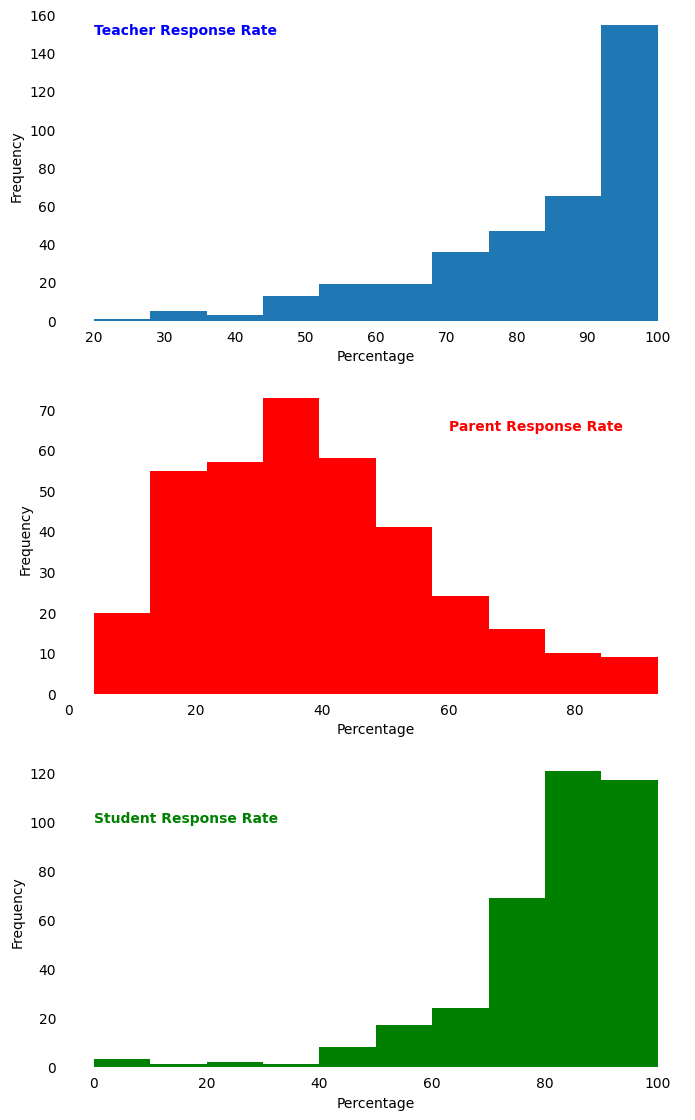Histogram plotted for response rate columns show the differences among the teacher, parent and student response rate to surveys. From the graph we can observe that for teachers and students histogram follows skew symmetry(right). Counts are higher above 90% and gradually decreases with decrease in percentage. Whereas for parents the histogram follows more or less symmetric curve (bell curve) with highest count at around 30% to 40% with counts decreasing both the sides but more gradual on the right side. This indicates that in most of the schools, teacher and student response is better (with 100% response rate) than the parent (with below 50% response rate) to surveys. Below we will plot the different survey results corresponding to teachers, parents and students. In : style.use('fivethirtyeight') fig, axs = plt.subplots(nrows=3, ncols=4, figsize=(14, 16)) axs[0,0].hist(combined['saf_t_11']) axs[0,1].hist(combined['com_t_11']) axs[0,2].hist(combined['eng_t_11']) axs[0,3].hist(combined['aca_t_11']) axs[1,0].hist(combined['saf_p_11'], color='red') axs[1,1].hist(combined['com_p_11'], color='red') axs[1,2].hist(combined['eng_p_11'], color='red') axs[1,3].hist(combined['aca_p_11'], color='red') axs[2,0].hist(combined['saf_s_11'], color='green') axs[2,1].hist(combined['com_s_11'], color='green') axs[2,2].hist(combined['eng_s_11'], color='green') axs[2,3].hist(combined['aca_s_11'], color='green') axs[2,0].set_xlabel('safety') axs[2,1].set_xlabel('communication') axs[2,2].set_xlabel('engagement') axs[2,3].set_xlabel('academic') # axs[0,0].set_ylabel('frequency') axs[1,0].set_ylabel('Frequency') # axs[2,0].set_ylabel('frequency') axs[0,0].set_title('Teacher', weight='bold', color='blue') axs[1,0].set_title('Parent',weight='bold', color='red') axs[2,0].set_title('Student',weight='bold', color='green')  Out: Text(0.5, 1.0, 'Student')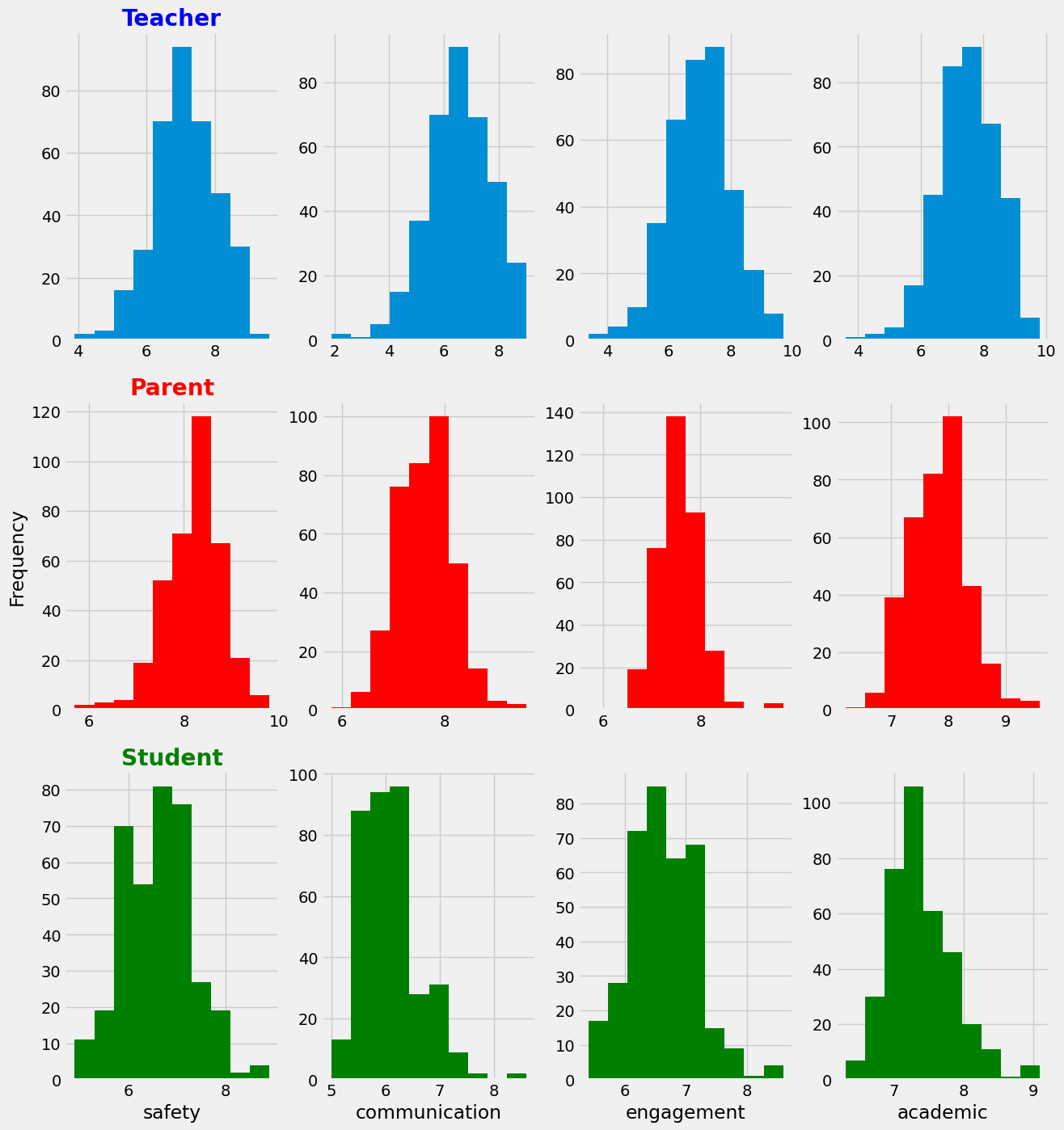In the above graph, first row, second row and third row represents teacher, parent and student surveys respectively. Whereas first, second, third and fourth column represents safety, communication, engagement and academic expectation surveys scores respectively. These surveys are ranked from 0 to 10. Below we will just mention the highest number of scores (frequency) marked by the teachers, parents and students. We can understand more about the distribution of these scores looking at the above graph. Safety Score- From the above graph we observe that highest number of teachers have marked safety score at 7, most parents have marked it at above 8 and most number of students marked safety in between 6 and 7. Communication Score- Highest number of teachers marked communication score at 7. Most parents have marked it at 8 and highest number students have marked communication at ~ 6. Engagement Score- Highest number of teachers marked engagement score at ~7. Parents have also marked it at ~7 and students have marked between 6 and 7. Academic Expectation Score- Highest number of teachers and parents have marked academic expectation score at 8. Students have marked at 7.5. Below we will calculate the average score of each category(among surveys) for teacher, parent and student. Make a plot and compare the results looking at the graph. In : #calculate the mean value for each category among surveys mean_rr = combined[['rr_t', 'rr_p', 'rr_s']].mean() mean_safety = combined[['saf_t_11', 'saf_p_11', 'saf_s_11']].mean() mean_com = combined[['com_t_11', 'com_p_11', 'com_s_11']].mean() mean_eng = combined[['eng_t_11', 'eng_p_11', 'eng_s_11']].mean() mean_acad = combined[['aca_t_11', 'aca_p_11', 'aca_s_11']].mean() #plot the above mean values fig, axs = plt.subplots(nrows=5, ncols=1, figsize=(10, 14)) axs.plot(mean_safety) axs.plot(mean_com, color='red') axs.plot(mean_eng, color='green') axs.plot(mean_acad, color='orange') axs.set_ylabel('Avg-Safety') axs.set_ylabel('Avg-Communication') axs.set_ylabel('Avg-Engagement') axs.set_ylabel('Avg-Academic') axs.plot(mean_rr, color='black') axs.set_ylabel('Avg-Response Rate')  Out: Text(0, 0.5, 'Avg-Response Rate')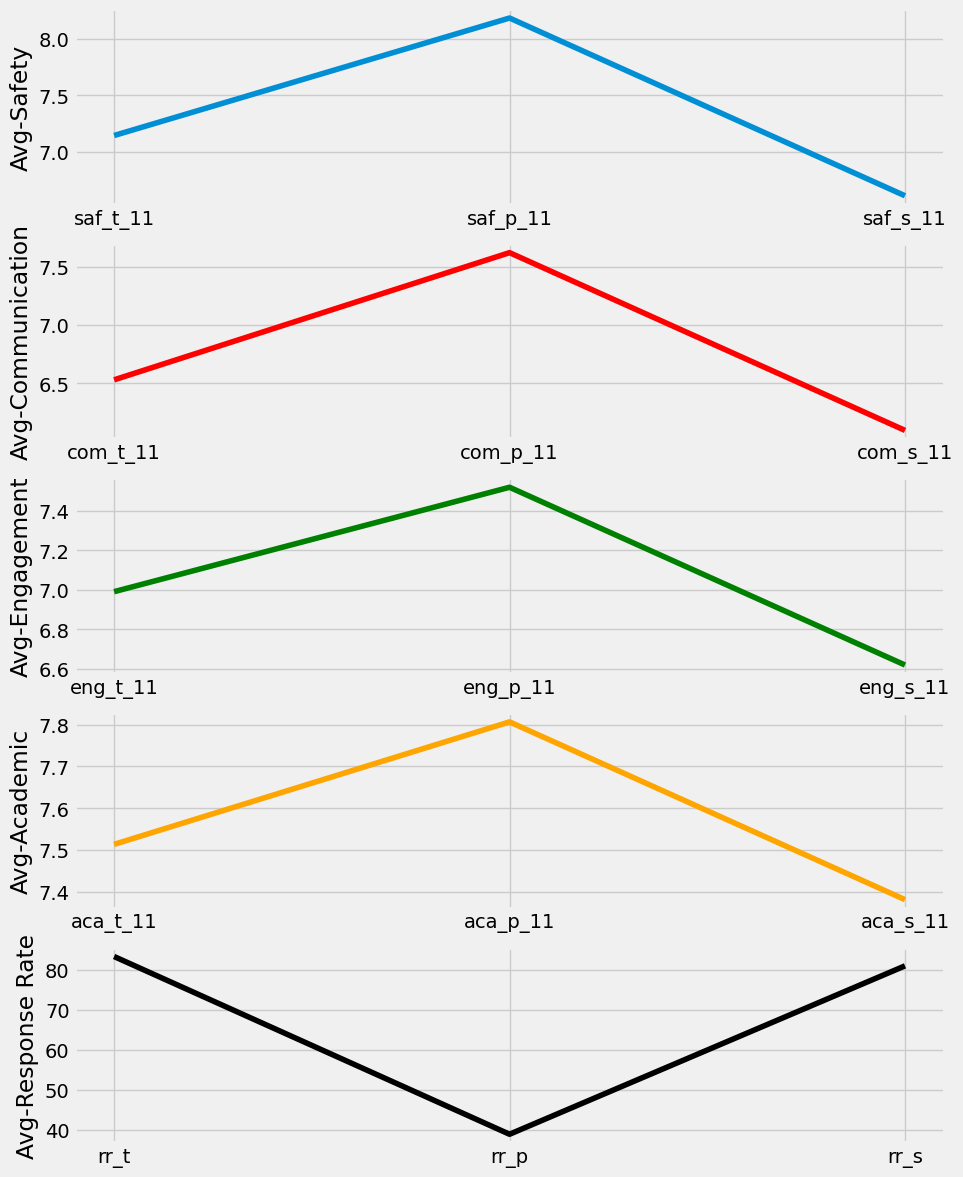From the above graph we observe that average survey scores for all the surveys is highest among parents. Second highest is teachers and the least is of students. But the average response rate for the surveys is lowest among parents in contrast with the different survey results. ## 11. Assigning Scores to Schools Based on SAT Score and Other Attributes¶ In this section we will assign scores to schools based on SAT Score, Total Safety (both in ascending order), and Property Price (in descending order). We will use df.sort_values() method for this purpose. In : #create a new dataframe by sorting the appropriate columns sort_sat = combined.sort_values(by=['sat_score', 'saf_tot_11', 'Price_$'], ascending=[False, False, True], ignore_index=True)
#set the index to begin with 1
sort_sat.index = sort_sat.index+1
#look at the few rows of the dataframe
sort_sat[['SCHOOL NAME', 'sat_score','saf_tot_11', 'Price_$']]  Out: SCHOOL NAME sat_score saf_tot_11 Price_$
1 STUYVESANT HIGH SCHOOL 2096.0 7.7 1084587.0
2 BRONX HIGH SCHOOL OF SCIENCE 1969.0 7.4 2094706.0
3 STATEN ISLAND TECHNICAL HIGH SCHOOL 1953.0 8.6 5107621.0
4 HIGH SCHOOL OF AMERICAN STUDIES AT LEHMAN COLLEGE 1920.0 8.7 2015222.0
5 TOWNSEND HARRIS HIGH SCHOOL 1910.0 8.2 4538714.0
... ... ... ... ...
359 INTERNATIONAL COMMUNITY HIGH SCHOOL 945.0 7.9 2000391.0
360 HIGH SCHOOL OF WORLD CULTURES 939.0 8.1 2027459.0
361 INTERNATIONAL SCHOOL FOR LIBERAL ARTS 934.0 7.8 2015241.0
362 INTERNATIONAL HIGH SCHOOL AT PROSPECT HEIGHTS 913.0 7.9 3029686.0
363 MULTICULTURAL HIGH SCHOOL 887.0 7.0 3090738.0

363 rows × 4 columns

The list above contains the School Names ranked from 1 till 363 (best to worst) based on the Sat Score, Safety and Property Price.

Below we list six best schools in the NewYork city based on good SAT Score (above 1700), better safety level (more than 7) and least expensive neighborhood (less than $1090000). In : best_school = combined[(combined['sat_score']>=1700) & (combined['saf_tot_11']>=7) & (combined['Price_$']<=1090000)]
best_school.index=numpy.arange(1, 7)
best_school[['SCHOOL NAME','sat_score', 'saf_tot_11', 'Price_$']]  Out: SCHOOL NAME sat_score saf_tot_11 Price_$
1 BARD HIGH SCHOOL EARLY COLLEGE 1856.0 8.5 1004062.0
2 ELEANOR ROOSEVELT HIGH SCHOOL 1758.0 8.5 1045949.0
3 STUYVESANT HIGH SCHOOL 2096.0 7.7 1084587.0
4 BEACON HIGH SCHOOL 1744.0 8.0 1030328.0
5 FIORELLO H. LAGUARDIA HIGH SCHOOL OF MUSIC & A... 1707.0 7.7 1030341.0
6 HIGH SCHOOL FOR MATHEMATICS, SCIENCE AND ENGIN... 1847.0 8.4 1084085.0

## Conclusions¶

In this project we analyzed SAT Scores along with the demographics of each New York City High Schools. The main focus of this project is to find out if demographics for e.g. gender, income, race etc. impacts the Sat Score. In the beginning we had total of seven different datasets. We cleaned them and made appropriate changes wherever necessary. At last we combined all seven of them on DBN column of each dataset to be left with a single, big dataframe. From the analysis of combined dataset we make following observations:-

• Analysis of Sat Score and Various Surveys:-

• In here we found out that with increase in student safety score there is a gradual increase in the SAT score. We have also calculated average total safety values for each of the five boroughs of New York city. This lead to the fact that Manhattan tops the list and Brooklyn is at the bottom of the list.

• We also found out that the average response rate of different surveys is lowest among parents compared to that of teachers and students (which are almost equal). But the average survey scores for different surveys is highest for parents. Next highest is for teachers and is last for students.

• Analysis of Sat Score and Race:- In here we found out that Sat score correlates positively with whites and asians with former being on top of the list. Blacks and hispanics correlate negatively with Sat score, with latter being at the bottom of the list. We observed an exponential decrease in Sat score with increase in hispanics percentage. There are 8 schools with >95% hispanics. In these schools atleast 80% of students are economically disadvantaged. These schools have very low state and national rankings. There are 5 schools with \<10% hispanics. In these schools minority enrolment is comparatively lower and lower percentage of economically disadvantaged students. These schools have very good state and national rankings.

• Analysis of Sat Score and Gender:- In here we found out that male percentage has a negative correlation and female percentage has a positive correlation with Sat Score. We did not find any pattern between sat score and female percentage but we came up with a list of 5 schools with >1700 sat score and >60% female percentage.

• We found an exponential increase in the Sat score with increase in the class size.

• We came up with a list of 6 neighborhoods with least property price i.e. \< \$1090000 and good sat score i.e. >1700.

• At last we ranked schools based on Sat scores, safety score (both best to least) and property price (lowest to highest).